The Sundial Primer created by Carl SabanskiThe Sundial Primer Index
 Horizontal Altitude Sundial Horizontal Altitude Sundial: an altitude dial in which hour lines for different dates are delineated on a horizontal surface. A vertical gnomon extends along the entire top end of the dial plate. If a vertical pole gnomon is used it is adjusted to the appropriate date. Latitude specific.Altitude Sundial: (or elevation dial): any dial which uses the sun's altitude, rather than its azimuth, for indicating the time. Usually does not need to be aligned N-S. Examples are ring dials, flag dials and shepherds' dials. Altitude dials were often incorporated in quadrants and folding rules.Altitude (of the sun) (a, ALT): the angular distance of the centre of the sun's disk above the observer's horizon (negative numbers indicate that the sun is below the horizon). It is measured along the principal plane to the sun's centre, and is the complement of the zenith distance. It is part of the horizontal co-ordinate system. The horizontal altitude sundial indicates the time by the sun's altitude, which depends on the latitude and the time of year. The sundial must be designed for a particular latitude. Figure 1 illustrates the dial. The hour lines appear as curved lines on the horizontal dial plate amongst the vertical date lines. The gnomon is either a vertical plate across the end of the dial plate or a vertical pin that can be moved so that it aligns with a particular day of the year. The date scale is inscribed below the gnomon. If moveable, once the gnomon is aligned with the appropriate date, the dial is held facing the sun and turned until the shadow is parallel to the date lines. The time can then be read. If the gnomon is a plate, the dial is held facing the sun and turned until the shadow is perpendicular to the date lines. The time can then be read.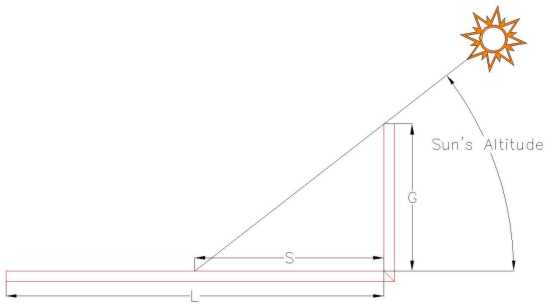Figure 1: Horizontal Altitude Sundial (CAD)A chart of the hour lines can be drawn on paper and attached to the dial plate or they may inscribed directly on the surface.As the sun's altitude reaches a lower value, early in the morning and later in the evening, the shadow cast by the gnomon becomes very long. By selecting a gnomon height G the shadow length S for the earliest and/or latest hour to be shown can be determined. This is done by using the equation provided below. The dial plate length L can then be established. Once the length is determined, a dial plate width can be selected.Knowing the dimensions of the chart, the width can be divided into 12  bands that represent the 12 months. Additional lines can be added to further divide the months into smaller periods.The position of the hour lines can now be determined. First the sun's altitude "a" must be determined for a given hour (Local Apparent Time) of a particular day. The distance S of the point on the hour line from the base of the chart is determined as follows:S = G / tan awhere G is the length of the gnomon and a is the altitude of the sun.Figure 2 illustrates the layout of the hour lines for a horizontal altitude dial at 30 minute intervals. As this dial indicates local apparent time (LAT), the hour lines are symmetrical about noon. The calculations need only be done for the morning hour lines as the afternoon hour lines are the same. This is because the sun's altitude is the same for equivalent time periods before and after noon (LAT), e.g. 8 am and 4 pm.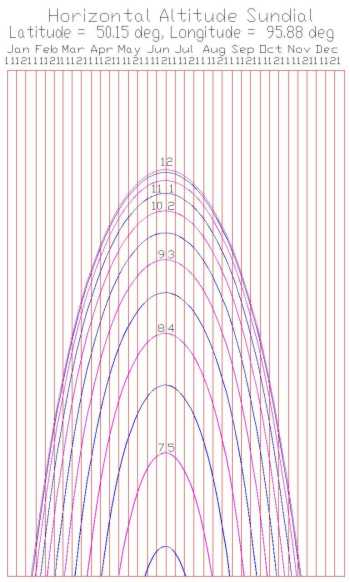Figure 2: Horizontal Altitude Sundial Hour Lines - Local Apparent Time (SONNE/CAD) From this figure it can be seen that the dial is least accurate around noon as the hour lines are very close together.It is possible to extend the range of time that the sundial will indicate by attaching a vertical altitude dial at the end of the horizontal altitude dial plate furthest away from the gnomon. Figure 3 illustrates this design.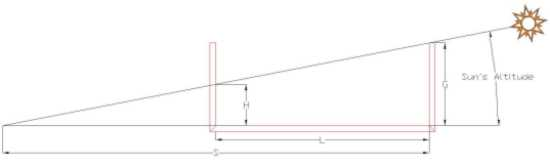Figure 3: Horizontal Altitude Sundial with Vertical Altitude Sundial (CAD)When the shadow length S exceeds the length L of the horizontal altitude sundial it will begin to move up the vertical altitude sundial. The distance H for any hour line, from the base of the vertical altitude dial, can be determined as follows:H = (S-L) tan aIt has been determined that "S = G / tan a" and as a result:H = G - (L tan a)Figure 4 illustrates the layout of the hour lines for a combined horizontal and vertical altitude sundial. This is the same sundial shown in Figure 2 but with the attached vertical altitude sundial. The date lines are continued from the horizontal altitude sundial into the vertical altitude sundial.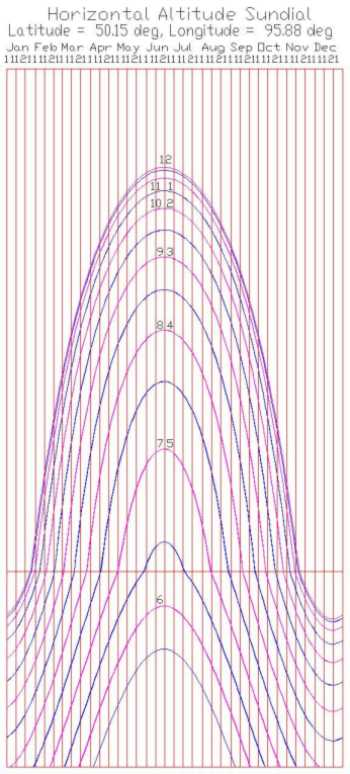Figure 4: Horizontal/Vertical Altitude Sundial Hour Lines - Local Apparent Time (SONNE/CAD)It is also possible to correct the hour lines for longitude and the Equation of Time.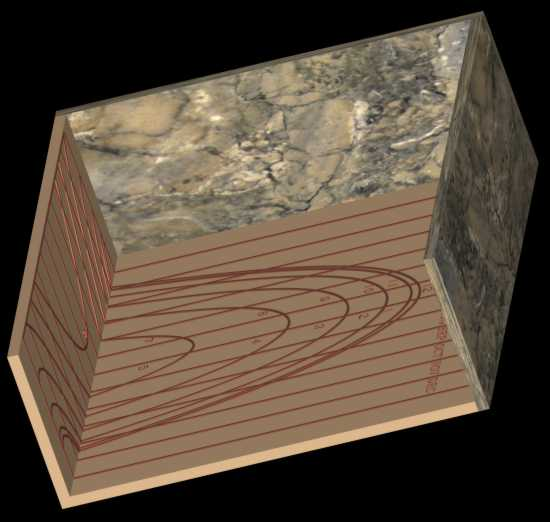For an image complete with shadow click here.